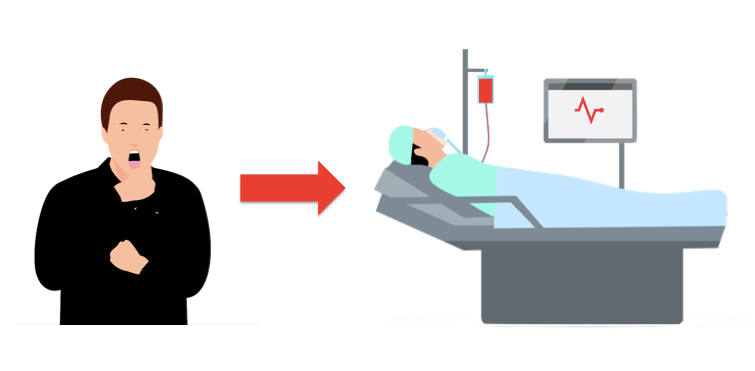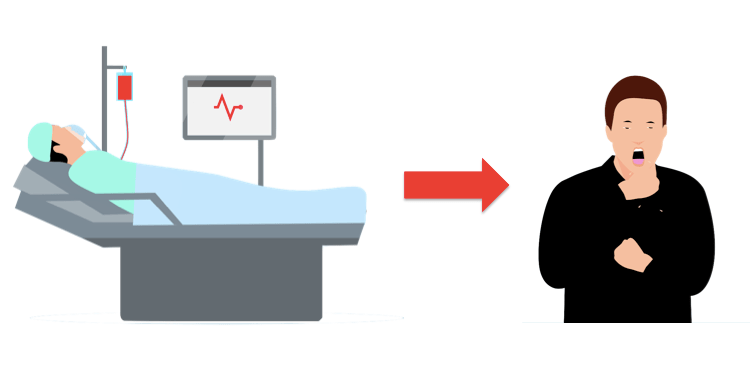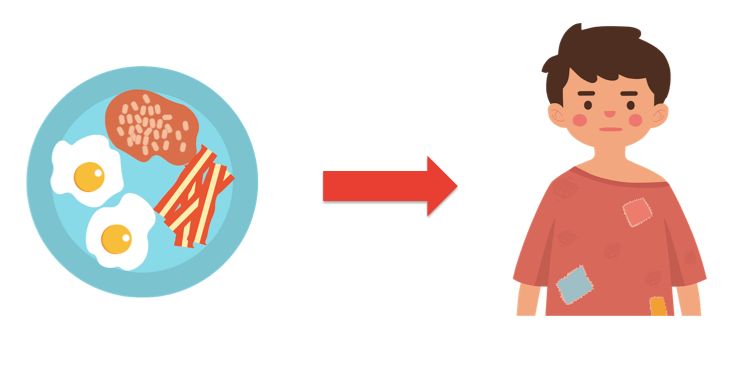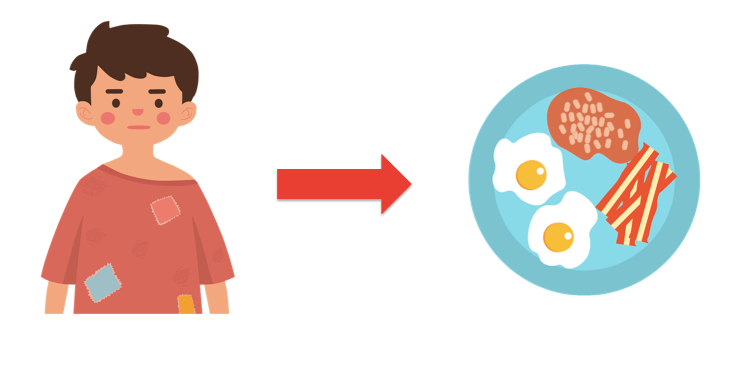# How to use cause and effect in a sentence

What is the cause?

Cause refers to why something happens.

What is the effect?

Effect refers to what happens as a result.

Ultimately the cause and effect is the relationship between two things in which one thing makes something else happen as a result. Understanding the cause and effect can help us to understand the relationships between things or events in a story. In a sentence, the cause can come first followed by the effect, or the effect can come first followed by the clause.

We can use signal words to help us identify the cause and effect.

Some examples of signal words that introduce the cause are:

➜ As a result of
➜ Because
➜ Since

Some examples of signal words that introduce the effect are:

➜ Hence
➜ So
➜ Therefore

Example 1

John was unwell, so he went to the hospital.
In this example, the cause comes first, followed by the effect. The signal word “so” helps us identify that the effect is being introduced.The sentence can also be written as: John went to the hospital because he was unwell.
In this example, the effect comes first, followed by the cause. The signal word “because” helps us identify that the cause is being introduced.Example 2

I didn’t eat breakfast; therefore, I am very hungry.
In this example, the cause comes first, followed by the effect. The signal word “therefore” helps us identify that the effect is being introduced.The sentence can also be written as: I am very hungry because I didn’t eat breakfast.
In this example, the effect comes first, followed by the cause. The signal word “because” helps us identify that the cause is being introduced.# How to use cause and effect in a sentence

What is the cause?

Cause refers to why something happens.

What is the effect?

Effect refers to what happens as a result.

Ultimately the cause and effect is the relationship between two things in which one thing makes something else happen as a result. Understanding the cause and effect can help us to understand the relationships between things or events in a story. In a sentence, the cause can come first followed by the effect, or the effect can come first followed by the clause.

We can use signal words to help us identify the cause and effect.

Some examples of signal words that introduce the cause are:

➜ As a result of
➜ Because
➜ Since

Some examples of signal words that introduce the effect are:

➜ Hence
➜ So
➜ Therefore

Example 1

John was unwell, so he went to the hospital.
In this example, the cause comes first, followed by the effect. The signal word “so” helps us identify that the effect is being introduced.

The sentence can also be written as: John went to the hospital because he was unwell.
In this example, the effect comes first, followed by the cause. The signal word “because” helps us identify that the cause is being introduced.

Example 2

I didn’t eat breakfast; therefore, I am very hungry.
In this example, the cause comes first, followed by the effect. The signal word “therefore” helps us identify that the effect is being introduced.

The sentence can also be written as: I am very hungry because I didn’t eat breakfast.
In this example, the effect comes first, followed by the cause. The signal word “because” helps us identify that the cause is being introduced.